# Topological structure (topology)

(diff) ← Older revision | Latest revision (diff) | Newer revision → (diff)

open topology, respectively, closed topology

A collection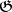, respectively, of subsets of a set, satisfying the following properties: 1) The set, as well as the empty set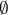, are elements of the collection, respectively., respectively. The intersection, respectively union, of a finite number, and the union, respectively intersection, of any number of elements of, respectively, is an element of the same collection.

Once a topology, or topological structure, has been introduced or defined on a set, the set is called a topological space, its elements are called points and the elements of the collection, respectively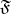, are called the open, respectively closed, sets of this topological space.

If one of the collectionsorof subsets ofis defined, satisfying property 1 and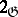or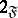, respectively, then the other collection may be defined by duality as consisting of the complements of the elements of the first collection.